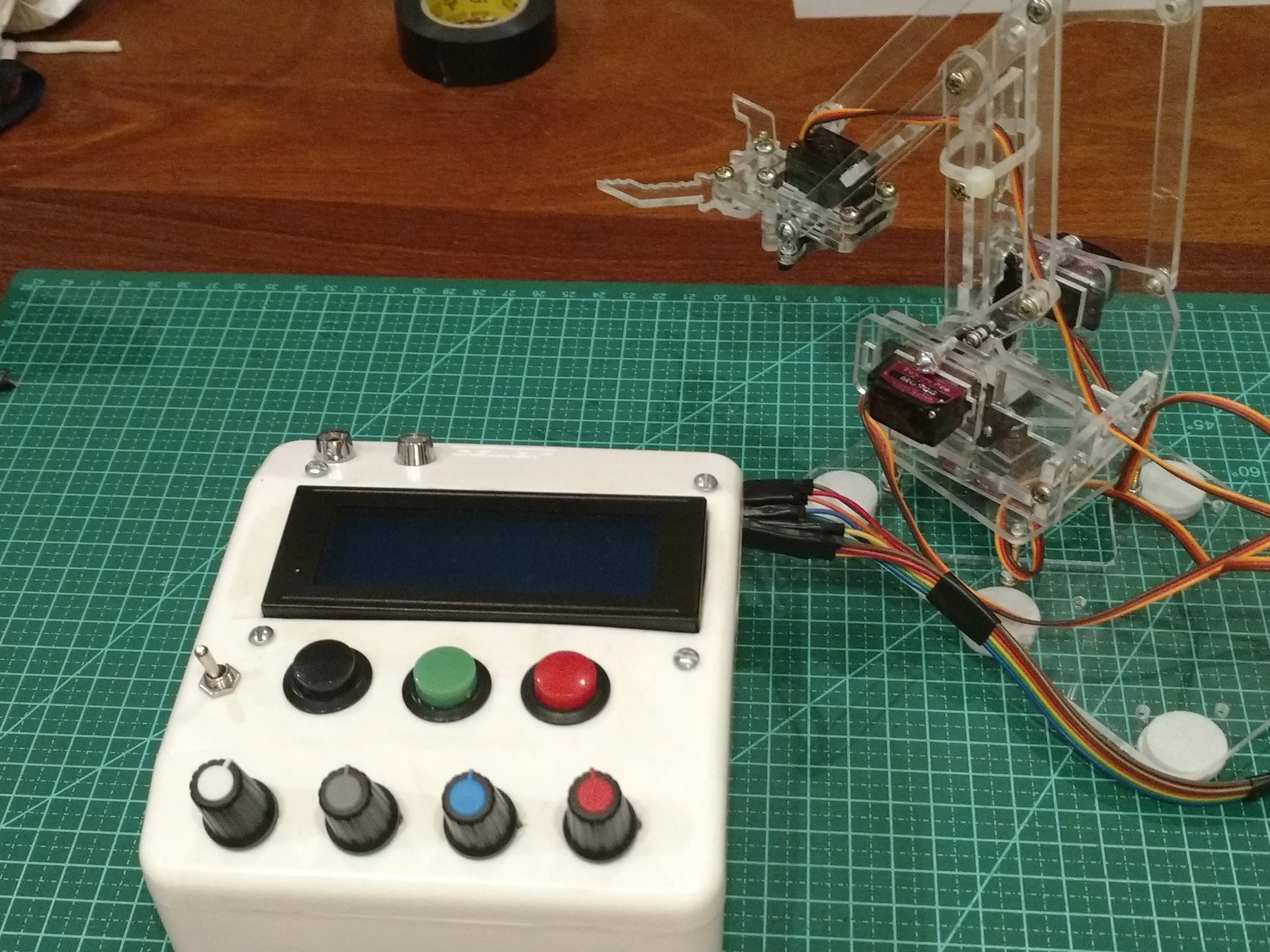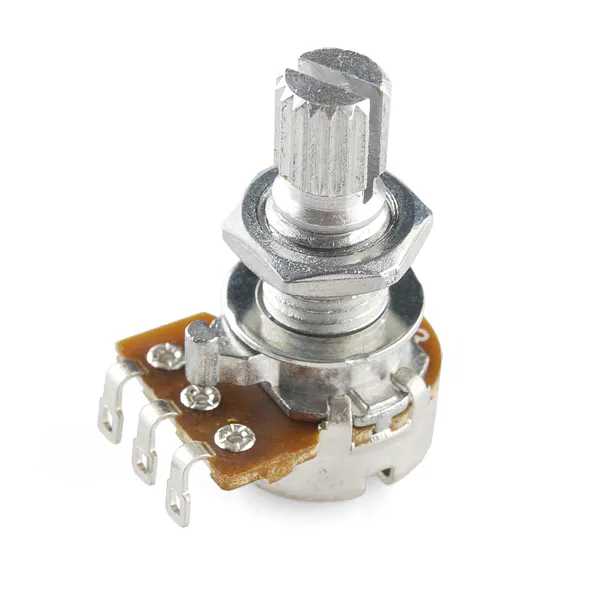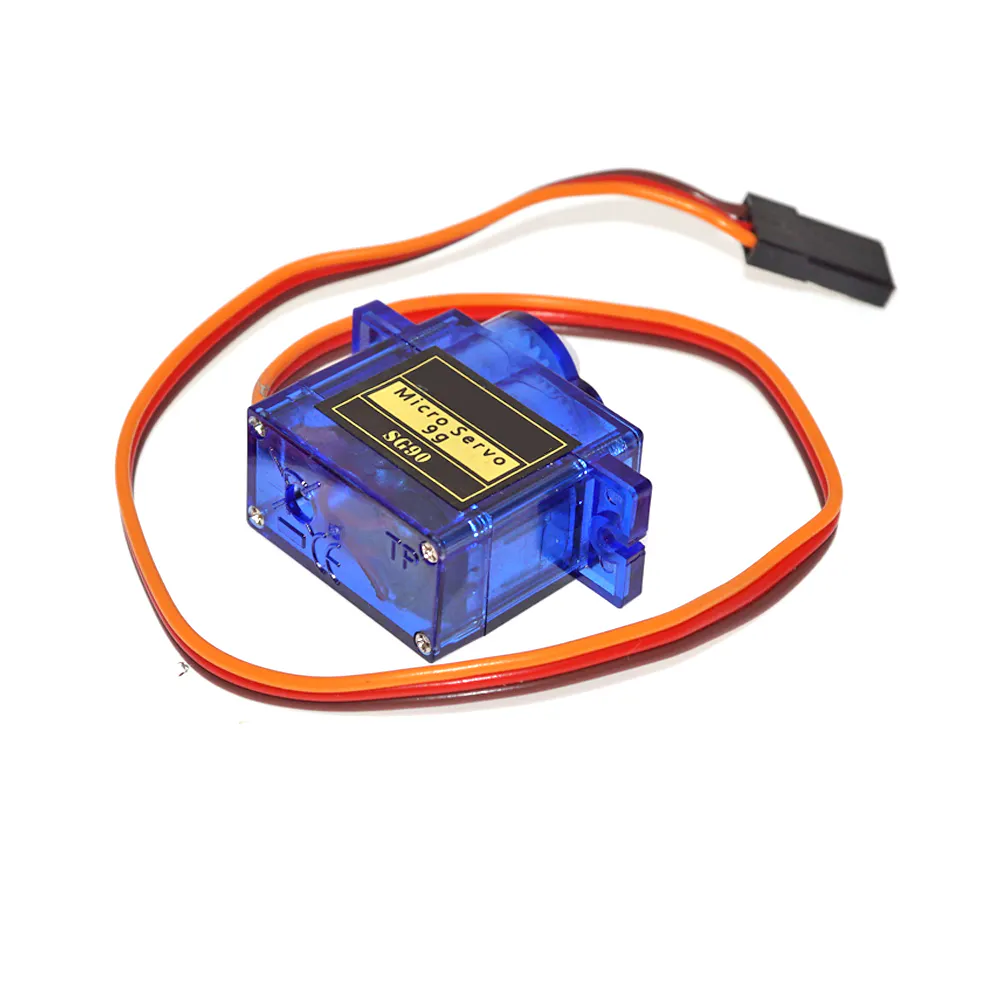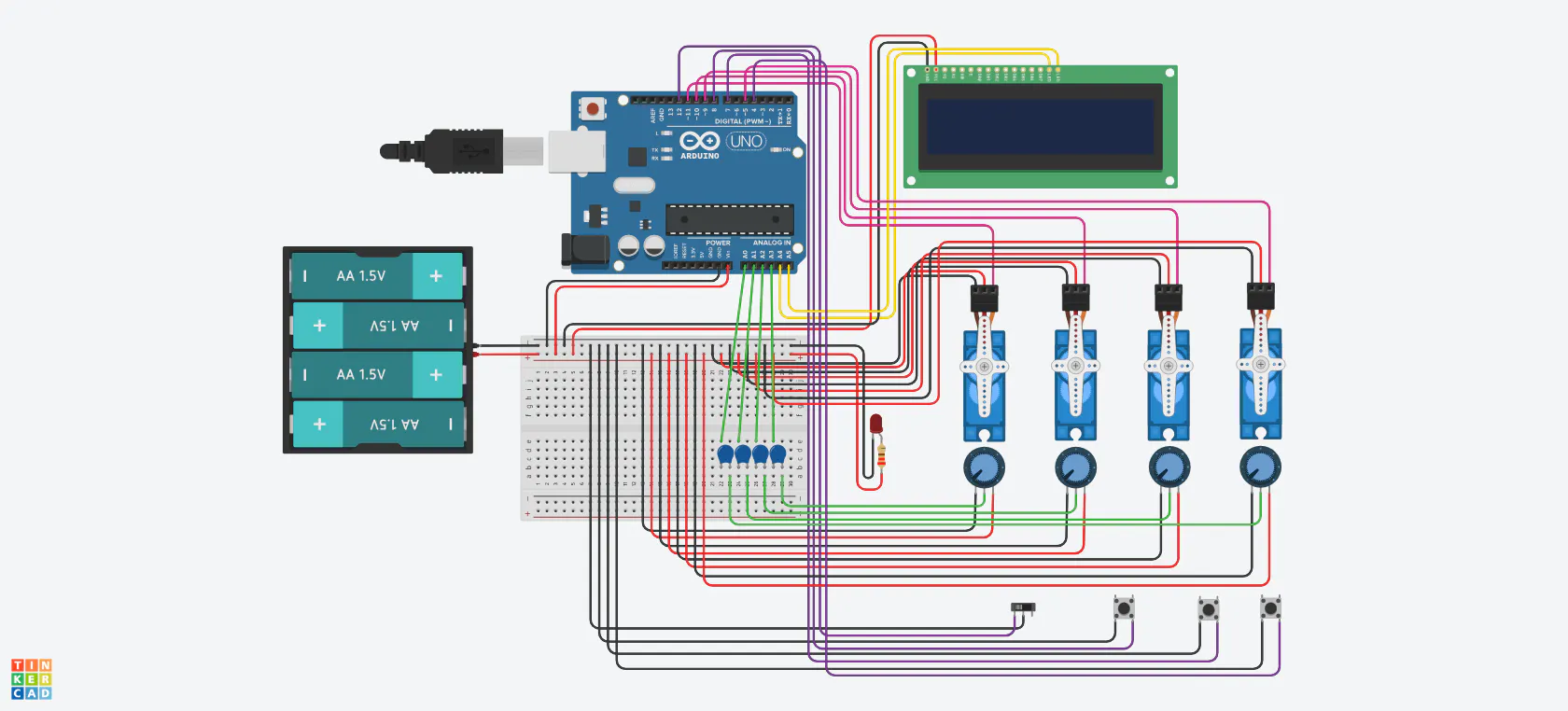Published © GPL3+

# Tobor, the Arm

Manual and programmable robotic arm with fancy control panel.

IntermediateShowcase (no instructions)3,580## Things used in this project

### Hardware componentsArduino UNO & Genuino UNO
×1
 20x4 Display with I2C module
×1Rotary potentiometer (generic) 10kohms
×4
 Colored push-button with colored heads
×3
 Switch button
×15 mm LED: Red
×1SG90 Micro-servo motor
×4Jumper wires (generic)
×1
 MeArm or any other 4DOF robotic arm
×1
 LED housing for a finished control panel
×1
 P4 female outlet for external power input
×1
 External Power source 5V/4A or 4 x 1,5V batteries to get 6V
×1

### Software apps and online servicesArduino IDE

### Hand tools and fabrication machinesSoldering iron (generic)
 Cutting pliers

Read more

## Schematics

### Scheme (Display connections are only to see the four wires

Tinkercad does no have the I2C 20x4 display. I use a 16x2 instead, with only 4 wires to represent 5V/GND and SCL/SDA## Code

### Tobor, the Arm

Arduino
Comment, Improve, Share
```/*
* 6/2/19Versao que funcionou com o control box. apos muitos problemas de mau contato e qualidade inferior
* dos servos azuis. Trocados por Towerpro MG90S engrenagem metal.
* iniciar montagem do braco MeArm
*/

#include <Wire.h>
#include <Servo.h>
#include <LiquidCrystal_I2C.h> // For 20x4 lcd
LiquidCrystal_I2C lcd(0x27,20,4);

Servo s1;  // create servo object to control a servo
Servo s2;
Servo s3;
Servo s4;

int potpin1 = A0;  // analog pin used to connect the potentiometer
int potpin2 = A1;  // analog pin used to connect the potentiometer
int potpin3 = A2;  // analog pin used to connect the potentiometer
int potpin4 = A3;  // analog pin used to connect the potentiometer

int valA = 0;    // read the value from the analog pin
int valB = 0;    // read the value from the analog pin
int valC = 0;    // read the value from the analog pin
int valD = 0;    // read the value from the analog pin

int greenButton = 12; //record mem index and servo angles
int redButton = 4; //reserved for future use
int blackButton = 7; //mem index selector
int switchAM = 8; //switch between Manual or Automatic operation
int counter = 0; //mem index
int mTime = 500;//time to move each servo in Aumtomatic mode one by one

//for now this is the way I found to store 10 * 4servo positions.
//An array of a mem counter and angles of 4 servos
int memP01, memP02, memP03, memP04, memP05, memP06, memP07, memP08, memP09, memP10;

//move ARM according to 4 angles stored in each Array
void moveCounter1() {
s1.write(memP01); delay(mTime);
s2.write(memP01); delay(mTime);
s3.write(memP01); delay(mTime);
s4.write(memP01); delay(mTime);
}

void moveCounter2() {
s1.write(memP01); delay(mTime);
s2.write(memP01); delay(mTime);
s3.write(memP01); delay(mTime);
s4.write(memP01); delay(mTime);
s1.write(memP02); delay(mTime);
s2.write(memP02); delay(mTime);
s3.write(memP02); delay(mTime);
s4.write(memP02); delay(mTime);
}

void moveCounter3() {
s1.write(memP01); delay(mTime);
s2.write(memP01); delay(mTime);
s3.write(memP01); delay(mTime);
s4.write(memP01); delay(mTime);
s1.write(memP02); delay(mTime);
s2.write(memP02); delay(mTime);
s3.write(memP02); delay(mTime);
s4.write(memP02); delay(mTime);
s1.write(memP03); delay(mTime);
s2.write(memP03); delay(mTime);
s3.write(memP03); delay(mTime);
s4.write(memP03); delay(mTime);
}

void moveCounter4() {
s1.write(memP01); delay(mTime);
s2.write(memP01); delay(mTime);
s3.write(memP01); delay(mTime);
s4.write(memP01); delay(mTime);
s1.write(memP02); delay(mTime);
s2.write(memP02); delay(mTime);
s3.write(memP02); delay(mTime);
s4.write(memP02); delay(mTime);
s1.write(memP03); delay(mTime);
s2.write(memP03); delay(mTime);
s3.write(memP03); delay(mTime);
s4.write(memP03); delay(mTime);
s1.write(memP04); delay(mTime);
s2.write(memP04); delay(mTime);
s3.write(memP04); delay(mTime);
s4.write(memP04); delay(mTime);
}

void moveCounter5() {
s1.write(memP01); delay(mTime);
s2.write(memP01); delay(mTime);
s3.write(memP01); delay(mTime);
s4.write(memP01); delay(mTime);
s1.write(memP02); delay(mTime);
s2.write(memP02); delay(mTime);
s3.write(memP02); delay(mTime);
s4.write(memP02); delay(mTime);
s1.write(memP03); delay(mTime);
s2.write(memP03); delay(mTime);
s3.write(memP03); delay(mTime);
s4.write(memP03); delay(mTime);
s1.write(memP04); delay(mTime);
s2.write(memP04); delay(mTime);
s3.write(memP04); delay(mTime);
s4.write(memP04); delay(mTime);
s1.write(memP05); delay(mTime);
s2.write(memP05); delay(mTime);
s3.write(memP05); delay(mTime);
s4.write(memP05); delay(mTime);
}

void moveCounter6() {
s1.write(memP01); delay(mTime);
s2.write(memP01); delay(mTime);
s3.write(memP01); delay(mTime);
s4.write(memP01); delay(mTime);
s1.write(memP02); delay(mTime);
s2.write(memP02); delay(mTime);
s3.write(memP02); delay(mTime);
s4.write(memP02); delay(mTime);
s1.write(memP03); delay(mTime);
s2.write(memP03); delay(mTime);
s3.write(memP03); delay(mTime);
s4.write(memP03); delay(mTime);
s1.write(memP04); delay(mTime);
s2.write(memP04); delay(mTime);
s3.write(memP04); delay(mTime);
s4.write(memP04); delay(mTime);
s1.write(memP05); delay(mTime);
s2.write(memP05); delay(mTime);
s3.write(memP05); delay(mTime);
s4.write(memP05); delay(mTime);
s1.write(memP06); delay(mTime);
s2.write(memP06); delay(mTime);
s3.write(memP06); delay(mTime);
s4.write(memP06); delay(mTime);
}

void moveCounter7() {
s1.write(memP01); delay(mTime);
s2.write(memP01); delay(mTime);
s3.write(memP01); delay(mTime);
s4.write(memP01); delay(mTime);
s1.write(memP02); delay(mTime);
s2.write(memP02); delay(mTime);
s3.write(memP02); delay(mTime);
s4.write(memP02); delay(mTime);
s1.write(memP03); delay(mTime);
s2.write(memP03); delay(mTime);
s3.write(memP03); delay(mTime);
s4.write(memP03); delay(mTime);
s1.write(memP04); delay(mTime);
s2.write(memP04); delay(mTime);
s3.write(memP04); delay(mTime);
s4.write(memP04); delay(mTime);
s1.write(memP05); delay(mTime);
s2.write(memP05); delay(mTime);
s3.write(memP05); delay(mTime);
s4.write(memP05); delay(mTime);
s1.write(memP06); delay(mTime);
s2.write(memP06); delay(mTime);
s3.write(memP06); delay(mTime);
s4.write(memP06); delay(mTime);
s1.write(memP07); delay(mTime);
s2.write(memP07); delay(mTime);
s3.write(memP07); delay(mTime);
s4.write(memP07); delay(mTime);
}

void moveCounter8() {
s1.write(memP01); delay(mTime);
s2.write(memP01); delay(mTime);
s3.write(memP01); delay(mTime);
s4.write(memP01); delay(mTime);
s1.write(memP02); delay(mTime);
s2.write(memP02); delay(mTime);
s3.write(memP02); delay(mTime);
s4.write(memP02); delay(mTime);
s1.write(memP03); delay(mTime);
s2.write(memP03); delay(mTime);
s3.write(memP03); delay(mTime);
s4.write(memP03); delay(mTime);
s1.write(memP04); delay(mTime);
s2.write(memP04); delay(mTime);
s3.write(memP04); delay(mTime);
s4.write(memP04); delay(mTime);
s1.write(memP05); delay(mTime);
s2.write(memP05); delay(mTime);
s3.write(memP05); delay(mTime);
s4.write(memP05); delay(mTime);
s1.write(memP06); delay(mTime);
s2.write(memP06); delay(mTime);
s3.write(memP06); delay(mTime);
s4.write(memP06); delay(mTime);
s1.write(memP07); delay(mTime);
s2.write(memP07); delay(mTime);
s3.write(memP07); delay(mTime);
s4.write(memP07); delay(mTime);
s1.write(memP08); delay(mTime);
s2.write(memP08); delay(mTime);
s3.write(memP08); delay(mTime);
s4.write(memP08); delay(mTime);
}

void moveCounter9() {
s1.write(memP01); delay(mTime);
s2.write(memP01); delay(mTime);
s3.write(memP01); delay(mTime);
s4.write(memP01); delay(mTime);
s1.write(memP02); delay(mTime);
s2.write(memP02); delay(mTime);
s3.write(memP02); delay(mTime);
s4.write(memP02); delay(mTime);
s1.write(memP03); delay(mTime);
s2.write(memP03); delay(mTime);
s3.write(memP03); delay(mTime);
s4.write(memP03); delay(mTime);
s1.write(memP04); delay(mTime);
s2.write(memP04); delay(mTime);
s3.write(memP04); delay(mTime);
s4.write(memP04); delay(mTime);
s1.write(memP05); delay(mTime);
s2.write(memP05); delay(mTime);
s3.write(memP05); delay(mTime);
s4.write(memP05); delay(mTime);
s1.write(memP06); delay(mTime);
s2.write(memP06); delay(mTime);
s3.write(memP06); delay(mTime);
s4.write(memP06); delay(mTime);
s1.write(memP07); delay(mTime);
s2.write(memP07); delay(mTime);
s3.write(memP07); delay(mTime);
s4.write(memP07); delay(mTime);
s1.write(memP08); delay(mTime);
s2.write(memP08); delay(mTime);
s3.write(memP08); delay(mTime);
s4.write(memP08); delay(mTime);
s1.write(memP09); delay(mTime);
s2.write(memP09); delay(mTime);
s3.write(memP09); delay(mTime);
s4.write(memP09); delay(mTime);
}

void moveCounter10() {
s1.write(memP01); delay(mTime);
s2.write(memP01); delay(mTime);
s3.write(memP01); delay(mTime);
s4.write(memP01); delay(mTime);
s1.write(memP02); delay(mTime);
s2.write(memP02); delay(mTime);
s3.write(memP02); delay(mTime);
s4.write(memP02); delay(mTime);
s1.write(memP03); delay(mTime);
s2.write(memP03); delay(mTime);
s3.write(memP03); delay(mTime);
s4.write(memP03); delay(mTime);
s1.write(memP04); delay(mTime);
s2.write(memP04); delay(mTime);
s3.write(memP04); delay(mTime);
s4.write(memP04); delay(mTime);
s1.write(memP05); delay(mTime);
s2.write(memP05); delay(mTime);
s3.write(memP05); delay(mTime);
s4.write(memP05); delay(mTime);
s1.write(memP06); delay(mTime);
s2.write(memP06); delay(mTime);
s3.write(memP06); delay(mTime);
s4.write(memP06); delay(mTime);
s1.write(memP07); delay(mTime);
s2.write(memP07); delay(mTime);
s3.write(memP07); delay(mTime);
s4.write(memP07); delay(mTime);
s1.write(memP08); delay(mTime);
s2.write(memP08); delay(mTime);
s3.write(memP08); delay(mTime);
s4.write(memP08); delay(mTime);
s1.write(memP09); delay(mTime);
s2.write(memP09); delay(mTime);
s3.write(memP09); delay(mTime);
s4.write(memP09); delay(mTime);
s1.write(memP10); delay(mTime);
s2.write(memP10); delay(mTime);
s3.write(memP10); delay(mTime);
s4.write(memP10); delay(mTime);
}
/*
for(int a = 0; a < 5; a++)
{
Serial.println(memP01[a]);
Serial.println(memP02[a]);
Serial.println(memP03[a]);
Serial.println(memP04[a]);
}
Serial.println("ln");
*/

void runningScreen() {
lcd.clear();
lcd.setCursor (0,0); lcd.print("PROGRAM      ");
lcd.setCursor (0,1); lcd.print("RUNNING >    ");
delay(100);
lcd.setCursor (0,1); lcd.print("RUNNING  >   ");
delay(100);
lcd.setCursor (0,1); lcd.print("RUNNING   >  ");
delay(100);
lcd.setCursor (0,1); lcd.print("RUNNING    > ");
delay(100);
lcd.setCursor (0,1); lcd.print("RUNNING      ");
delay(100);
}

void setup() {

// no anoying phisical  resistors to be welded...I hope it works
pinMode(switchAM, INPUT_PULLUP);
pinMode(blackButton, INPUT_PULLUP);
pinMode(greenButton, INPUT_PULLUP);
pinMode(redButton, INPUT_PULLUP);

Serial.begin(9600); // do not need this...the ctrlbox have a lcd.
lcd.init();
lcd.backlight();
// lcd.begin(16, 2);
lcd.setCursor(0, 0); lcd.print("ROBOTIC ARM");
lcd.setCursor(0, 1); lcd.print("VERSION 001");
delay(3000);
// attaches the PWM pins to servos
s1.attach(11);
s2.attach(10);
s3.attach(9);
s4.attach(6);

} //end setup

void loop() {

int switchAMState = digitalRead(switchAM);

if (switchAMState == 0){ // Manual Mode
lcd.clear();
lcd.setCursor(0, 0);
lcd.print("MANUAL OPERATION");

valA = analogRead(potpin1);            // reads the value of the potentiometer (value between 0 and 1023)
valA = map(valA, 1, 1023, 180,0);     //values acheived from testing to avoid motor creep at pot end courses
s1.write(valA);
delay(15);
lcd.setCursor(0, 1);
lcd.print("S1: ");
lcd.print(valA); //show servo angle on lcd

valB = analogRead(potpin2);
valB = map(valB, 1, 1023, 180,0);
s2.write(valB);
delay(15);
lcd.setCursor(10, 1);
lcd.print("S2: ");
lcd.print(valB);

valC = analogRead(potpin3);
valC = map(valC, 1, 1023, 0, 180);
s3.write(valC);
delay(15);
lcd.setCursor(0,3);
lcd.print("S3: ");
lcd.print(valC);

valD = analogRead(potpin4);    // reads the value of the potentiometer (value between 0 and 1023)
valD = map(valD, -25, 1023, 0, 180);     // calibrating to avois creeping

//calibration to let maximum claw opening of 90degrees and pressure excess on claws.dont know if its useful.
if (valD > 40 || valD < 170) {
s4.write(valD);
delay(15);
lcd.setCursor(10, 3);
lcd.print("S4: ");
lcd.print(valD);
}
else {
valD = 41;
}

delay(100);

int  greenButtonState = digitalRead(greenButton);
if (greenButtonState == 0) {
counter = counter +1;

switch (counter) {

case 1:
memP01 = counter; // save counter
memP01 = valA;
memP01 = valB;
memP01 = valC;
memP01 = valD;
break;

case 2:
memP02 = counter; // save counter
memP02 = valA; memP02 = valB; memP02 = valC; memP02 = valD;
break;

case 3:
memP03 = counter; // save counter
memP03 = valA; memP03 = valB; memP03 = valC; memP03 = valD;
break;

case 4:
memP04 = counter; // save counter
memP04 = valA; memP04 = valB; memP04 = valC; memP04 = valD;
break;

case 5:
memP05 = counter; // save counter
memP05 = valA; memP05 = valB; memP05 = valC; memP05 = valD;
break;

case 6:
memP06 = counter; // save counter
memP06 = valA; memP06 = valB; memP06 = valC; memP06 = valD;
break;

case 7:
memP07 = counter; // save counter
memP07 = valA; memP07 = valB; memP07 = valC; memP07 = valD;
break;

case 8:
memP08 = counter; // save counter
memP08 = valA; memP08 = valB; memP08 = valC; memP08 = valD;
break;

case 9:
memP09 = counter; // save counter
memP09 = valA; memP09 = valB; memP09 = valC; memP09 = valD;
break;

case 10:
memP10 = counter; // save counter
memP10 = valA; memP10 = valB; memP10 = valC; memP10 = valD;
break;

case 11:
counter =1;

}//end switch

lcd.clear();
lcd.setCursor(0,0); lcd.print("ARM POSITION ");
lcd.setCursor(0,1); lcd.print("RECORDED     ");
lcd.setCursor(0,2); lcd.print("P  S1  S2  S3  S4");
lcd.setCursor(0,3); lcd.print(counter);
lcd.setCursor(4,3); lcd.print(valA);
lcd.setCursor(8,3); lcd.print(valB);
lcd.setCursor(12,3); lcd.print(valC);
lcd.setCursor(16,3); lcd.print(valD);
delay(2000);
} //endgreenbt

//if needed to correct some  arm position
int blackButtonState = digitalRead(blackButton);
if (blackButtonState == 0) {
if (counter <10){ counter = counter + 1; }
else { counter = 1; }
lcd.clear();
lcd.setCursor(0,0); lcd.print("MEM POSITION: ");
lcd.setCursor(8,1); lcd.print(counter);
delay(1000);
}//end ifblackbt

}//end if switchAM

else { //set ARM to Automatic Mode

if (counter > 0){
switch (counter){

case 1:
moveCounter1();
break;
case 2:
moveCounter2();
break;

case 3:
moveCounter3();
break;

case 4:
moveCounter4();
break;

case 5:
moveCounter5();
break;

case 6:
moveCounter6();
break;

case 7:
moveCounter7();
break;

case 8:
moveCounter8();
break;

case 9:
moveCounter9();
break;

case 10:
moveCounter10();
break;
} //end switch

runningScreen();

} //end if counter>0

else {
lcd.clear();
lcd.setCursor(0,0);
lcd.print("EMPTY MEMORY!");
delay(2000);

} // end else if counter

} //end else switchAM key

}   //end loop
```

## Credits

### NerdFatherRJ

2 projects • 18 followers